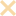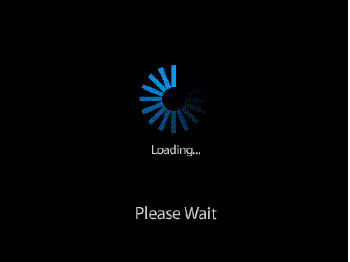APPOr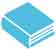OG20官方指南，建议同学们刷3遍。第一遍做题了解题型和考点，第二遍精刷，第三遍集中解决疑难问题。建议考生第一遍刷题采用官方正版纸质书籍，若遇到疑难问题，欢迎在此专区查阅解析，提供解析，参与题目讨论，与所有考生一起解决疑难问题。

##### 请描述一下这个错误：

【OG20-P287-386题】

On the number line, point R has coordinate r and point T has coordinate t. Is t < 0 ?

(1) -1 < r< 0

(2) The distance between R and T is equal to r2

• 分析A选项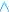xxxxxxxxxxxxxxxxxxxxxxxxxxxxxxxxxxxx
• 分析B选项xxxxxxxxxxxxxxxxxxxxxxxxxxxxxxxxxxxx
• 分析C选项xxxxxxxxxxxxxxxxxxxxxxxxxxxxxxxxxxxx
• 分析D选项xxxxxxxxxxxxxxxxxxxxxxxxxxxxxxxxxxxx
• 分析E选项xxxxxxxxxxxxxxxxxxxxxxxxxxxxxxxxxxxx暂无雷哥网文字解析

`条件1：不充分条件2：不充分1+2：|r^2|<|r|→t<0，充分`

### 题目讨论 （10条评论）•你这只猪

```画图直接列举数字，-1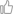0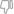0

回复

2020-06-08 14:04:01

```
•cindyGMAT330

`这样讲， 当-1 t=-(r^2 + r ) 那t就是负数; 另外 coordinate是坐标的意思 数字线上的坐标 应该就是指数字吧。 开始我看到coordinate也纠结了好久 xy数轴的话 根本没法思考了。。。`00 回复 2019-11-21 16:47:21
•cindyGMAT330

`这样讲， 当-1 -t=r^2 + r => t=-(r^2 + r ) 那t就是负数; 另外 coordinate是坐标的意思 数字线上的坐标 应该就是指数字吧。 开始我看到coordinate也纠结了好久 xy数轴的话 根本没法思考了。。。`00 回复 2019-11-21 16:46:10
•cindyGMAT330回复cindyGMAT330

为什么我打字不能完整。。。 奇怪00 回复 2019-11-21 16:47:50

•波波lll

`这道题在说什么……没有一个单词不认识却看不懂`00 回复 2019-11-05 16:23:21
•想养一只博美

```条件1：-10,有r-t=r^2,即t=r(1-r)，已知条件1有 -10，有t-r=r^2,即t=r(1+r),条件1有-100

回复

2019-10-24 13:37:37

```
•`是有图的吗。。。`00 回复 2019-07-20 16:12:19
•Jess123

`1+2：f-r=r2，f=r2+r>0充分`00 回复 2019-03-31 16:47:51
•ziyuanguo

`什么意思啊`00 回复 2019-01-28 18:02:22
•驴子旋呀

```bb
h
Statement Two Alone:

The distance between R and T is equal to r^2.

The distance between two values on the number line is the absolute value of the difference between the two values. Thus statement two gives us the equation |r - t| = r^2. However, without knowing anything about r and t, we can’t determine whether t is less than zero. For instance, r could be 2 and t could be -2; or r could be -2 and t could be 2. In each of the cases, |r - t| = 4 = r^2; but in one case t > 0 and in the other t < 0. Statement two is not sufficient to answer the question. We can eliminate answer choice B.

Statements One and Two Together:

Using statements one and two, we know that r is a negative proper fraction and |r - t| = r^2. Thus, r - t = r^2 OR r - t = -r^2. Solving each of these for t, we get: t = r - r^2 OR t = r + r^2.

Since r is a negative proper fraction, no matter what the value of r is, t will always be a negative number. For instance, if r = -1/2, then r^2 = 1/4 and t will either be -1/2 - 1/4 = -3/4 or -1/2 + 1/4 = -1/4.

The reason why t cannot be positive is that when we square r (a negative proper fraction), the value of r^2 (though positive) will be less than the absolute value of r. Recall that t = r - r^2 or t = r + r^2. When a positive proper fraction with a smaller absolute value is added to (or subtracted from) a negative proper fraction with a larger absolute value, the sum (or difference) will always be less than zero.

https://gmatclub.com/forum/on-the-number-line-point-r-has-coordinate-r-and-point-t-has-coordinat-220426.html```00 回复 2018-10-19 14:22:00
•phylliskong38

`在左边，r-t=r^2， t<0 在右边，t-r=r^2, t<0 所以联立t<0,充足`00 回复 2018-08-28 22:54:09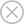• 手机注册
• 邮箱注册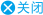###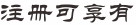•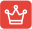#### 知识库学习

GMAT语法、逻辑、阅读、数学各单项备考知识点学习及测验
•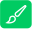#### 在线做题

包含GMAT各单项必考知识点题目、OG/PREP/GWD/雷哥讲义题目、难度650/680/700/730题库题目练习及题目解析
•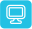#### 在线模考

语文套题/数学套题/全套仿真模考，包含GWD/PERP/精选模考等上百套套题模考
•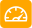#### 在线测评

适合5种不同基础的GMAT学员，测评后可自动出具分数报告及复习计划指导
•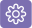#### 资料下载

GMAT必备备考资料下载、鸡精下载、课程课件等免费下载
•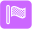#### 课程学习

注册会员后，可在GMAT课程区，选择免费直播课程及公开课程进行在线学习
×